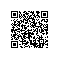# 用深度学习来获取文本语义: 词向量应用于自然语言处理◆ ◆

W(“woman”)−W(“man”) ≃ W(“queen”)−W(“king”)#### CBOW模型#### 连续跳跃元语法模型#### 代码

（你可以在这个GitHub库里找到下面例子的代码）

#### 从维基百科上找一个大数据集

• 找到你想处理的语言的ISO 639代码：ISO 639代码的列表

• 登录https://dumps.wikimedia.org/wiki/latest/ （译者注：此链接已失效）

• 下载wiki-latest-pages-articles.xml.bz2

pip install –upgrade gensim

from gensim.corpora import WikiCorpus

language_code = “he”

inp = language_code+”wiki-latest-pages-articles.xml.bz2″

outp = “wiki.{}.text”.format(language_code)

i = 0

print(“Starting to create wiki corpus”)

output = open(outp, ‘w’)

space = ” ”

wiki = WikiCorpus(inp, lemmatize=False, dictionary={})

for text in wiki.get_texts():

article = space.join([t.decode(“utf-8”) for t in text])

output.write(article + “\n”)

i = i + 1

if (i % 1000 == 0):

print(“Saved ” + str(i) + ” articles”)

output.close()

print(“Finished – Saved ” + str(i) + ” articles”)

#### 训练模型

• size：向量的维度

• 大的size值会要求更多的训练数据，但能带来更准确的模型

• window：在一个句子内，目标词与预测词之间的最大距离

• min_count：忽略所有总词频低于这个值的词。

import multiprocessing

from gensim.models import Word2Vec

from gensim.models.word2vec import LineSentence

language_code = “he”

inp = “wiki.{}.text”.format(language_code)

out_model = “wiki.{}.word2vec.model”.format(language_code)

size = 100

window = 5

min_count = 5

start = time.time()

model = Word2Vec(LineSentence(inp), sg = 0, # 0=CBOW , 1= SkipGram

size=size, window=window, min_count=min_count, workers=multiprocessing.cpu_count())

# trim unneeded model memory = use (much) less RAM

model.init_sims(replace=True)

print(time.time()-start)

model.save(out_model)

#### fastText库

pip install fasttext

start = time.time()

language_code = “he”

inp = “wiki.{}.text”.format(language_code)

output = “wiki.{}.fasttext.model”.format(language_code)

model = fasttext.cbow(inp,output)

print(time.time()-start)

#### 评估向量：类比性

W(“woman”) ≃ W(“man”)+ W(“queen”)− W(“king”)

import numpy as np

from gensim.matutils import unitvec

def test(model,positive,negative,test_words):

mean = []

for pos_word in positive:

mean.append(1.0 * np.array(model[pos_word]))

for neg_word in negative:

mean.append(-1.0 * np.array(model[neg_word]))

# compute the weighted average of all words

mean = unitvec(np.array(mean).mean(axis=0))

scores = {}

for word in test_words:

if word not in positive + negative:

test_word = unitvec(np.array(model[word]))

# Cosine Similarity

scores[word] = np.dot(test_word, mean)

print(sorted(scores, key=scores.get, reverse=True)[:1])

positive_words = [“queen”,”man”]

negative_words = [“king”]

# Test Word2vec

print(“Testing Word2vec”)

model = word2vec.getModel()

test(model,positive_words,negative_words,model.vocab)

# Test Fasttext

print(“Testing Fasttext”)

model = fasttxt.getModel()

test(model,positive_words,negative_words,model.words)

#### 结果

Testing Word2vec

[‘woman’]

Testing Fasttext

[‘woman’]

W(“woman”) ≃ W(“man”)+ W(“queen”)− W(“king”)使用钉钉扫一扫加入圈子
+ 订阅